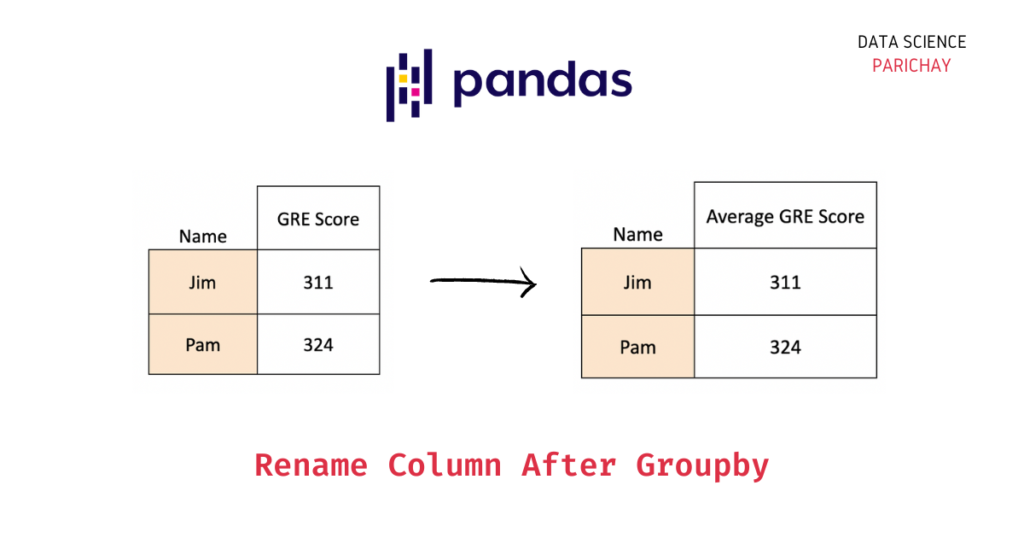# Pandas – Rename Columns in Dataframe after Groupby

You can use the following steps to rename columns after the groupby operation on a pandas dataframe.

1. Group the dataframe on the desired column (for example, “col1”) with the desired aggregation (for example, mean of “col2”).
2. Use the pandas dataframe `rename()` function to change the name of “col2” to your desired new name (for example, “avg_col2”).

Note that if you try to change the name of the grouping column (“col1”), you’ll get an error. This is because the resulting dataframe after groupby doesn’t have the grouping column (“col1”), its unique values are used as the index in the dataframe. If you still want it as a separate column, apply the `reset_index()` function and then use the `rename()` function to rename this column.

## Example – Change column name after groupby in Pandas

Let’s take a look at a step-by-step example.

First, we will create a dataframe that we will be using throughout this tutorial.

```import pandas as pd

# create a dataframe of GRE scores of two students
df = pd.DataFrame({
'Name': ['Jim', 'Jim', 'Jim', 'Pam', 'Pam'],
'Attempt': ['First', 'Second', 'Third', 'First', 'Second'],
'GRE Score': [298, 321, 314, 318, 330]
})
# display the dataframe
df```

Output:

Here, we created a dataframe of the GRE test scores of some students across their multiple attempts at the exam.

Let’s now group the above dataframe on “Name” to see the average score of each candidate.

```# group dataframe on "Name" and get the mean "GRE Score"
df.groupby("Name").agg({"GRE Score": "mean"})```

Output:

📚 Data Science Programs By Skill Level

Introductory

Intermediate ⭐⭐⭐

🔎 Find Data Science Programs 👨‍💻 111,889 already enrolled

Disclaimer: Data Science Parichay is reader supported. When you purchase a course through a link on this site, we may earn a small commission at no additional cost to you. Earned commissions help support this website and its team of writers.

We get a dataframe with the unique values from the “Name” column as the index and their respective mean “GRE Score” values in the “GRE Score” column.

Now, let’s change the name of the “GRE Score” column to “Average GRE Score” using the pandas dataframe `rename()` function.

```# group dataframe on "Name" and get the mean "GRE Score" and rename "GRE Score" to "Average GRE Score"
df.groupby("Name").agg({"GRE Score": "mean"}).rename(columns={"GRE Score": "Average GRE Score"})```

Output:

You can see that the aggregate column now is called the “Average GRE Score”.

Alternatively, you can directly specify the name of the resulting aggregate column inside the `.agg()` function.

```# group dataframe on "Name" and get the mean "GRE Score" with the column name "Average GRE Score"
df.groupby("Name").agg(average_gre_score=("GRE Score", "mean"))```

Output:

Note that here we had to use the column name with variable naming conventions.

You can similarly use multiple aggregations as well.

`df.groupby("Name").agg(attempts=("GRE Score", "count"), average_gre_score=("GRE Score", "mean"))`

Output:

Note that in both the above methods, we are changing the names of the columns resulting from aggregations and not the grouping column. This is because the grouping column does not exist in the resulting grouped dataframe. Its unique values are used as the dataframe’s index.

If you need those values as a separate column, use the `reset_index()` function. And then, if you further need to rename that column, use the `rename()` function.

Let’s look at an example.

`df.groupby("Name").agg({"GRE Score": "mean"}).reset_index().rename(columns={"Name": "Candidate", "GRE Score": "Average GRE Score"})`

Output:

Here, we group the data on “Name” to get the mean “GRE Score”, we then reset the index of the dataframe which creates the “Name” column from the dataframe index, and then we rename the “Name” column to “Candidate” and the “GRE Score” column to “Average GRE Score” using the pandas dataframe `rename()` function.

You might also be interested in –

•